1. Error Components - Random Effects - Variance Components
3.1 The Problem
Quite often we have disaggregated data for a large number of individuals (a cross section), say N, observed over a number of periods (a time series), say T. The problem is to combine the NT observations in order to make our estimator more efficient. We will explore issues of unbiasedness, consistency and efficiency in the context of pooled cross section -- time series data. We will begin with the random effects model since, arguably, the dummy variables model is a special case (or is it the other way around?).

The classic paper in applied economics is Nerlove's essay on the demand for natural gas. Most of the subsequent theoretical developments can be attributed to Swamy and Mehta. Other more recent names to look for are Baltagi and Avery.

Before going into details, a simple example (or choose the *.mcd file) will help you visualize what is going on.

For an R-script with output and a LIMDEP output that both deal with random effects models skip down ot the bottom of this page.

3.2 Set Up

There are i=1,...,N individuals observed over t=1,...,T periods and we posit the relationship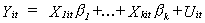(1)

When we have the same number of observations for each person the experimental design is known as a balanced block design. When the blocks are unequal all of the follwoing results hold, but only after some corrections.

Depending on the circumstance, we might also represent the model in one of two alternate forms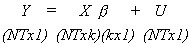or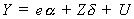where e is an NTx1 vector of ones, a is the corresponding intercept, Z are the remaining k-1 columns of X and d are slope coefficients.

Note the following:
1) We presume that all individuals have the same response to changes in the independent variables. The coefficients to be estimated are equal across persons and periods. That is, they all have the same MPC in a consumption model.

2) We might think that there are random effects across individuals. That is, at all points in time, a change in an unobserved variable affects each individual differently, but the effect is fixed over all the periods. Also, there may be a random effect in each period that affects all individuals in the same way. Finally, there may be unobservables that are random through time and across individuals.

Thus, we can decompose our error term as follows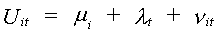mi represents the individual effects

lt represents the time effect

nit represents the purely random or white noise effect

We make the following assumptions about the components of the error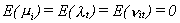That is, each component of the error term has a mean of zero.

The variance of the individual effect is the same for all persons, although the realization of the disturbance may differ across persons. Further, there is no correlation between persons.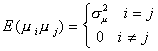The variance of the time effect is the same for all periods, but the realization differs from period to period. There is no serial correlation. If the time subscript does not match then the expectation is zero.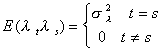The expectation of the white noise product is non zero only when both the individual and time subscripts match.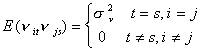Let us construct the covariance matrix for the population disturbance. We'll do this one piece at a time, starting with the individual effect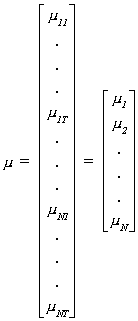Given our assumption about the individual random effect, we can write the covariance matrix for the ith person's random effect as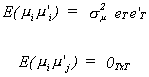eT is a column vector of ones of dimension Tx1. When we stack all of the individuals one over the other, the pattern of individual effects variances is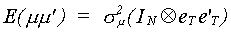This is a great big matrix of dimension NTxNT. There are a total of N blocks on the main diagonal, each of which is TxT. Each block is filled with the common variance. Every other position is filled with a zero.

Similarly for the time effect, we first stack the observations, running first through the time subscript then incrementing the individual subscript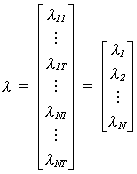Consider first the time effect covariance between person i and person j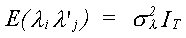This is a scalar diagonal matrix because the time subscripts match between the ith and jth persons.
For any given person we have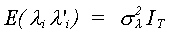Putting everyone back together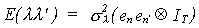This NTxNT matrix is composed of TxT scalar diagonal matrices everywhere.
Consider now the white noise term.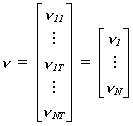Anywhere that both the individual and time subscripts match we get a nonzero expectation.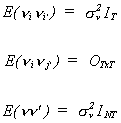The result is a big, NTxNT, scalar diagonal matrix.

Putting together the three pieces we get the patterned matrix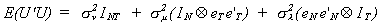1. Estimation

Let us begin by redefining some variances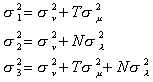The strategy that we employ is to go through the data the first time to obtain sets of residuals which are then used to construct estimates of the parameters in the error covariance matrix. As a strategy, this is similar to what you did to correct for autocorrelation using the Cochrane Orcutt technique.

Step 1.a

Construct a cross section regression by finding the mean of T observations for each individual. That is,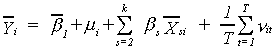Notice several things: We have shifted the position of the disturbance mi and that Slt = 0. One way to interpret this formulation would be to assert that the intercept is random. Parenthetically, P.A.V.B. Swamy extended this notion to make all of the regression coeffcients random.
The estimator applied to this equation is known as the between estimator and is equivalent to applying least squares to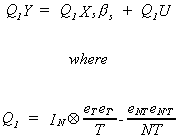Q1 is a matrix which puts the data in a form that provides one data point per person; we have only N observations now. Q1 is idempotent and has a trace of N-1. Each person's time mean is measured as a deviation from the grand mean. The result is that we lose the intercept. Hence, Xs refers to the set of independent variables excluding the column of ones for the intercept. An equivalent OLS formulation would be to use the original data, but include a dummy variable for each distinct person (no intercept then). Suppose that you have a cross section of time series on wages, the dependent variable, and schooling, the independent variable. Ability is an omitted variable that serves to shift the intercept across individuals. The question is whether the shift is random or fixed? You specify a random effects model. The result is that there will be a correlation between schooling and the error term. The testing procedure for discriminating between the least squares dummy variable model and the random effects model exploits this fact.
Let us look at the residual sum of squares for the 'between' estimator.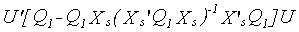Taking the trace and expected value we have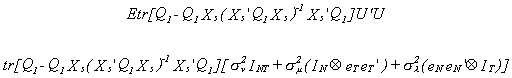Let us consider the term involving sl2. We are particularly interested in the product of Q1 and the Kronecker product term.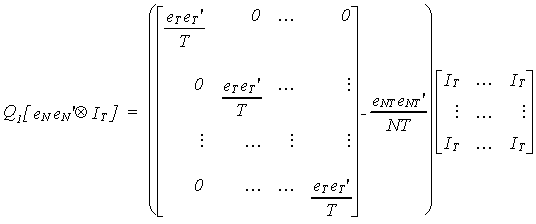with some manipulation we can show that this is zero so the sl2 term drops out.
Now consider the sm2 term, the relevant portion of which we reproduce here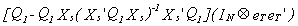Let us look specifically at the part involving the product of Q1 and the Kronecker product.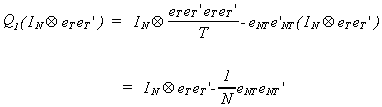The trace of this we can see to be NT - T, or T(N-1).
We also want to take advantage of the following property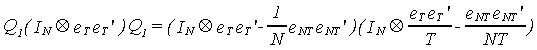If you multiply through by T/T you can see that we get TQ1Q1. Since Q1 is idempotent this is just TQ1. Therefore, when we put together some of the pieces involving the sm2 we can write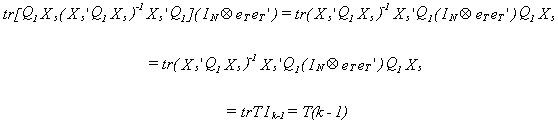Therefore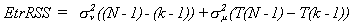So, using the residuals from the 'between' estimator,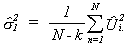is an unbiased estimator of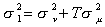. In the above equation the . indicates that we have already summed out the time effects

Step 1.b
Construct a time series regression by finding the mean of N observations at each point in time. That is,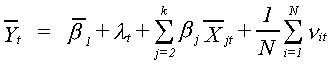Applying least squares to this equation is the 'within' estimator encountered in the analysis of variance. This estimation equation could be written as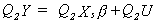where Q2 is given by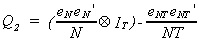Each observation is measured as a deviation of the mean across individuals for the tth period from the grand mean. The OLS equivalent to the 'within' estimator would be one which included a dummy variable for each time period (no intercept in that case).
Notice that we have shifted the position of the disturbance lt so that it is closely associated with the intercept. Again there is the question of whether the appropriate model is fixed effects or random effects. If we incorrectly specify the RE, then the error term will be correlated with the RHS variables. Also, Smi = 0. Note that the intercept can again be thought of as a random term with non-zero mean. From this regression we can save the residual sum of squares to construct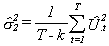which we can show to be unbiased using the same methods as applied in step 1.a. The . indicates that we have summed out the individual effects.

Step 1.c

We will now use time, state, and overall means to construct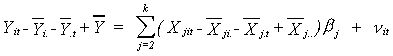There is no intercept in this model. It is equivalent to applying least squares to a model that has all the variables measured in their levels, but which has a set of dummies for individuals and a set of dummies for time periods. It is sometimes referred to as the least squares dummy variables model or, in ANOVA, the fully saturated model. The algebraic form taken by the saturated model is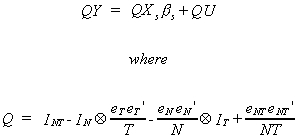Save the residuals from this model and construct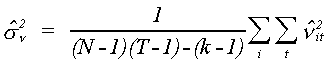which is also unbiased.

Step 2.a

Form the coefficients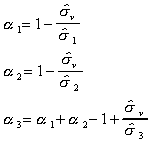where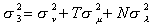.

Step 2.b

Construct the transformed variables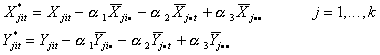Step 2.c

Now you are ready to estimate the parameters of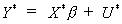3.4 Properties of the Estimator

We will consider two step estimators in general, of which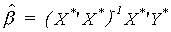is a particular example.

THEOREM

Consider the modelwe can regard this as a set of N equations with each equation having T observations. Assume that the disturbances in the different equations U1(t), ..., UN(t) follow a t-dimensional continuous probability law, symmetric about zero. That is, f(U1(t), ..., UN(t)) is an even function.
Then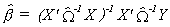where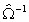is an unbiased estimator of W-1, is itself unbiased. Also assume U has a fourth moment and E(1-r-w)-1 exists.

Proof:

In part a. of the proof we show that the expectation of the estimator exists and in part b. we show that the estimator is unbiased.

a. Let h denote any vector of real numbers from NT dimensional space and consider the expectation of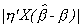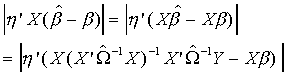Recall Y = X b + U, so we will make this substitution also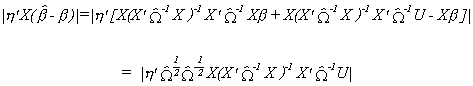Recall the Cauchy Schwartz Inequality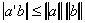For our problem we will adopt the following definitions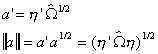and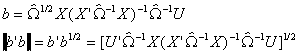Substituting into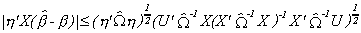Recall from our unit on linear algebra that if A-B is positive semi definite then Z'(A-B)Z 0. For our problem we'll let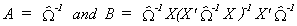So upon taking the difference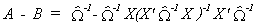Factoring the square root of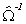out of this expression gives us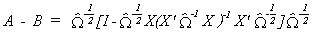The part in square brackets is idempotent so must be positive semi-definite. The square root of the inverse of the error covariance estimator,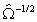, is also positive semi definite. Therefore A - B is positive semi definite and we can conclude that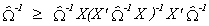and that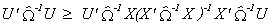Therefore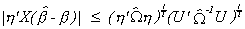Now introduce the following definitionsso that for the model as stated at the start of the theorem we can write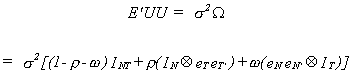The largest and smallest characteristic roots of the estimated error covariance matrix are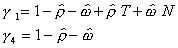From two theorems of linear algebra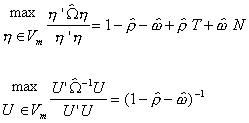Substituting these results into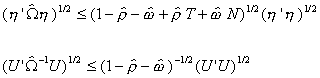Finally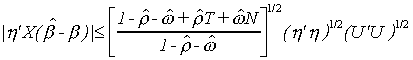Since all three terms on the right are finite we can conclude that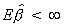1. is a continuous random variable since U is continuous. Therefore the probability that is singular is zero. We wish to demonstrate that E(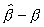)=0.
We can write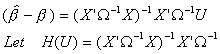Note that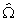is an even function of U, and therefore H(U) is also even. Now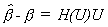is an odd function and is isomorphic about zero. So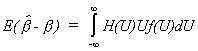H(U) and f(U) are even, U is odd, so the integrand is odd. Therefore, E()=0.

3.5 Testing the Specification
A. Random Effects vs. OLS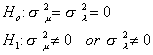Our test statistic will be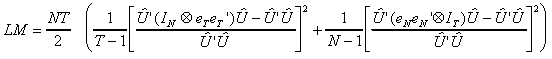where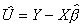. That is, the set of residuals is saved from applying OLS to the whole sample. In this case our test statistic is distributed as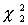.
We note the following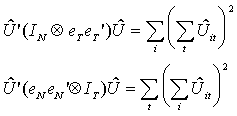The first of these sums the OLS residuals over time for each individual and squares the n results, then adds them up. The sum can be thought of as an estimate of the numerator of s12=su2+Tsm2. The second sums the OLS residuals over individuals for each period and squares the T results, then adds them up. The sum can be thought of as an estimate of the numerator of s22=su2+Tsl2. Under the null hypothesis sm2 and sl2 are both zero, so the terms in square brackets in the test statistic are zero.

B. Fixed Effects vs. OLS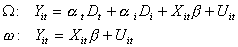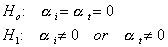The test statistic is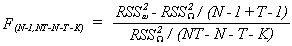C. Random Effects vs Least Squares Dummy Variable Model

1. The REM assumes that, for example, individual effects are uncorrelated with the other regressors. In the example provided earlier wages were regressed on schooling and we acknowledged that ability was an unobservable that could serve to shift the intercept. Now, if we had data on the entire population then LSDV would surely be the appropriate model. But since we are drawing only a sample REM might be appropriate. That is, the intercept varies in a random fashion across individuals due to sampling. The problem is that the random effect attributable to ability might be correlated with schooling.

2. If the random effects are correlated with other regressors then the random effects estimator is inconsistent due to omitted variables. Recall we raised this possibility of specification error earlier in the discussion.

Ho: No correlation. LSDV and REM are both consistent, LSDV is not efficient. Therefore the REM is the better estimator.

H1: Correlation. REM is not consistent, so use LSDV.

Under Ho LSDV and REM will not differ systematically, so we look at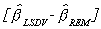We know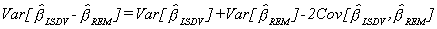A result due to Hausman is the following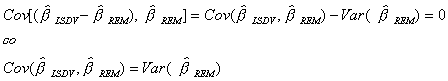Using this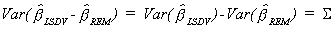Note that the variances in S exclude any terms corresponding to dummy variables and intercepts. It is based solely on the slope coefficients.
A Wald statistic is then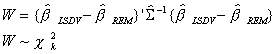An example is provided in another section of the lecture notes.

_______________________________________________________________________

Random Effects, R and LIMDEP

_______________________________________________________________________

The R-script is

## Example of Random Effects model from Venables and Ripley, page 205
library("nlme")
library("regress")
data(Oats)
names(Oats) <- c("B","V","N","Y")
Oats\$N <- as.factor(Oats\$N)

## Using regress
oats.reg <- regress(Y~N+V,~B+I(B:V),identity=TRUE,print.level=1,data=Oats)
summary(oats.reg)

## Using lme
oats.lme <- lme(Y~N+V,random=~1|B/V,data=Oats,method="REML")
summary(oats.lme)

The corresponding output for the REGRESS command is

Maximised Residual Log Likelihood is -214.975

Linear Coefficients:
Estimate     Std. Error
(Intercept)     79.917        8.220
N0.2              19.500        4.250
N0.4              34.833        4.250
N0.6              44.000        4.250
VMarvellous   5.292        7.079
VVictory        -6.875        7.079

Variance Coefficients:
Estimate  Std. Error
B           214.477  168.834
I(B:V)   109.693    67.711
I            162.559    32.191

The corresponding output for the LME command is

Linear mixed-effects model fit by REML
Data: Oats
AIC            BIC           logLik
586.0688   605.7756   -284.0344

Random effects:
Formula: ~1 | B
(Intercept)
StdDev: 14.64549        # 14.64549^2 = 214.477

Formula: ~1 | V %in% B
(Intercept)   Residual
StdDev:   10.47060    12.75034    # 10.4706^2 = 109.693 and 12.75034^2 = 162.559

Fixed effects: Y ~ N + V
Value     Std.Error  DF     t-value    p-value
(Intercept)     79.91667   8.219989   51    9.722235  0.0000
N0.2              19.50000   4.250113   51    4.588114  0.0000
N0.4              34.83333   4.250113   51    8.195861   0.0000
N0.6              44.00000   4.250113   51  10.352667   0.0000
VMarvellous   5.29167   7.077578   10    0.747666   0.4719
VVictory        -6.87500   7.077578   10   -0.971378   0.3543

Correlation:
(Intr)   N0.2    N0.4    N0.6   VMrvll
N0.2                -0.259
N0.4                -0.259   0.500
N0.6                -0.259   0.500   0.500
VMarvellous   -0.431   0.000   0.000   0.000
VVictory         -0.431   0.000   0.000    0.000 0.500

Standardized Within-Group Residuals:
Min               Q1                    Med          Q3               Max
-1.84137227 -0.66274193 -0.06682795 0.63830229 1.66054158

Number of Observations: 72
Number of Groups:
B     V %in% B
6            18

Output from LIMDEP

Fixed Effects

+----------------------------------------------------+
| Ordinary    least squares regression               |
| Model was estimated Jan 19, 2006 at 10:04:23AM     |
| LHS=YIELD    Mean                 =   103.9722     |
|              Standard deviation   =   27.05913     |
| WTS=none     Number of observs.   =         72     |
| Model size   Parameters           =          6     |
|              Degrees of freedom   =         66     |
| Residuals    Sum of squares       =   30179.08     |
|              Standard error of e  =   21.38361     |
| Fit          R-squared            =   .4194761     |
|              Adjusted R-squared   =   .3754970     |
| Model test   F[  5,    66] (prob) =   9.54 (.0000) |
| Diagnostic   Log likelihood       =  -319.5402     |
|              Restricted(b=0)      =  -339.1178     |
|              Chi-sq [  5]  (prob) =  39.16 (.0000) |
| Info criter. LogAmemiya Prd. Crt. =   6.205292     |
|              Akaike Info. Criter. =   6.204905     |
| Autocorrel   Durbin-Watson Stat.  =   .8174727     |
|              Rho = cor[e,e(-1)]   =   .5912637     |
+----------------------------------------------------+

+---------+--------------+----------------+--------+---------+----------+
|Variable | Coefficient  | Standard Error |t-ratio |P[|T|>t] | Mean of X|
+---------+--------------+----------------+--------+---------+----------+
Constant      79.9166667     6.17291691    12.946   .0000
N2            19.5000000     7.12787048     2.736   .0080     .25000000
N3            34.8333333     7.12787048     4.887   .0000     .25000000
N4            44.0000000     7.12787048     6.173   .0000     .25000000
V1           -6.87500000     6.17291691    -1.114   .2694     .33333333
V3            5.29166667     6.17291691      .857   .3944     .33333333

+--------------------------------------------------+
| Random Effects Model: v(i,t) = e(i,t) + u(i)     |
| Estimates:  Var[e]              =   .255399D+03  |
|             Var[u]              =   .214681D+03  |
|             Corr[v(i,t),v(i,s)] =   .456689      |
| Lagrange Multiplier Test vs. Model (3) =   80.50 |
| ( 1 df, prob value =  .000000)                   |
| (High values of LM favor FEM/REM over CR model.) |
| Baltagi-Li form of LM Statistic =          80.50 |
+--------------------------------------------------+

+---------+--------------+----------------+--------+---------+----------+
|Variable | Coefficient  | Standard Error |b/St.Er.|P[|Z|>z] | Mean of X|
+---------+--------------+----------------+--------+---------+----------+
N2            19.5000000     5.32707415     3.661   .0003     .25000000
N3            34.8333333     5.32707415     6.539   .0000     .25000000
N4            44.0000000     5.32707415     8.260   .0000     .25000000
Constant      79.3888889     7.06887251    11.231   .0000

Notice that Greene's Var(u) is quite close to the Variance due to the Block effect in R|REGRESS.  For this data set LIMDEP's estimator was not able to find positive estimates of the variances when there were random BLOCK and Variety effects.